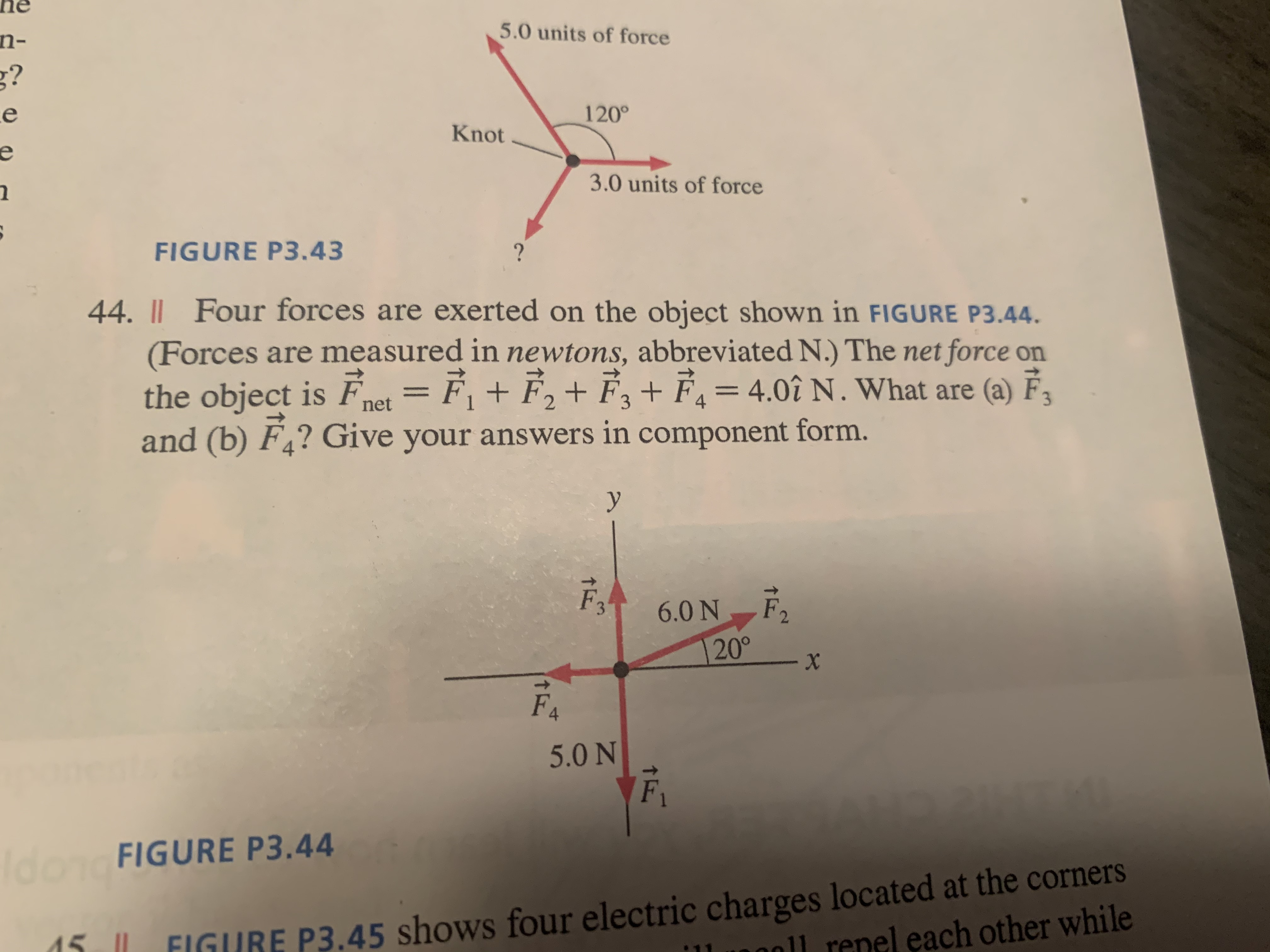# hen-5.0 units of forceg?120°Knote3.0 units of forceFIGURE P3.43?44. || Four forces are exerted on the object shown in FIGURE P3.44.(Forces are measured in newtons, abbreviated N.) The net force onthe object is Fnet = F¡ + F, + F,+ F, = 4.0î N. What are (a) F,and (b) F,? Give your answers in component form.%3D6.0 N F,20°X-F45.0 NIdon FIGURE P3.44P3.45 shows four electric charges located at the cornersnel each other while

Question
1 views

#44help_outlineImage Transcriptionclosehe n- 5.0 units of force g? 120° Knot e 3.0 units of force FIGURE P3.43 ? 44. || Four forces are exerted on the object shown in FIGURE P3.44. (Forces are measured in newtons, abbreviated N.) The net force on the object is Fnet = F¡ + F, + F,+ F, = 4.0î N. What are (a) F, and (b) F,? Give your answers in component form. %3D 6.0 N F, 20° X- F4 5.0 N Idon FIGURE P3.44 P3.45 shows four electric charges located at the corners nel each other while fullscreen
check_circle

Step 1

Given information:

The resultant of all the forces (Fr) = 4i N

The magnitude of the force (F1) = -5j N (-ve y direction)

The magnitude of the force (F2) = 6 N at direction which makes 200 with +ve x-axis.

= 6.Cos(200) i + 6.Sin(200) j

= 5.63 N i + 2.05 N j

Step 2

From the diagram we can say that the vertical forces are balancing each other hence we can write:

F3 + F2sin(20) + F1 = 0

F3 = 5 N - 2.05 N = 2.95 N j

Step 3

Since the resultant is in “+ve” x-direction we can write the force balance equation as follows:

F2...

### Want to see the full answer?

See Solution

#### Want to see this answer and more?

Solutions are written by subject experts who are available 24/7. Questions are typically answered within 1 hour.*

See Solution
*Response times may vary by subject and question.
Tagged in

### Physics